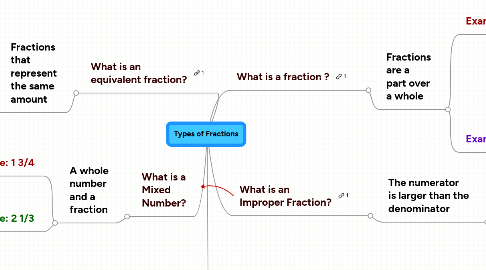# Types of Fractions

Get Started. It's FreeTypes of Fractions## 1. What is a fraction ?

### 1.1. Fractions are a part over a whole

1.1.1. Example: 1/2

1.1.1.1. The part is called the NUMERATOR in this fraction we are talking about 1 part.

1.1.1.2. The DENOMINATOR is the whole. In this fraction it is the number 2. It tells how many pieces the whole is divided into.

1.1.2. Example: 3/5

1.1.2.1. The part is called the Numerator. In this fraction the numerator is the number 3.

1.1.2.2. The DENOMINATOR is the whole. In this fraction the whole is divided into 5 parts.

## 2. What is an Improper Fraction?

### 2.1. The numerator is larger than the denominator

2.1.1. Example: 7/4

2.1.1.1. The numerator has 7 parts and and the denominator is cut into fourths.

2.1.2. Example: 7/3

2.1.2.1. The Numerator has 7 parts, the Denominator is cut into thirds

## 3. What is an equivalent fraction?

### 3.1. Fractions that represent the same amount

3.1.1. Example: 2/4

3.1.1.1. 2/4 = 4/8

3.1.2. Example: 6/10

3.1.2.1. 6/10= 12/20

## 4. What is a Mixed Number?

### 4.1. A whole number and a fraction

4.1.1. Example: 1 3/4

4.1.1.1. This mixed number has 1 whole and the fraction 3/4.

4.1.2. Example: 2 1/3

4.1.2.1. This mixed number has 2 wholes and the fraction 1/3